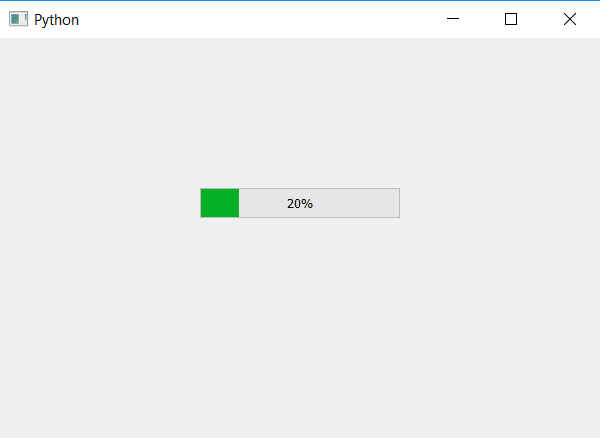Open In App

# PyQt5 – How to set the maximum value of progress bar ?

In this article we will see how to set the maximum value of progress bar. By default the maximum value of progress bar is 100, but we can change it to our need. In order to do this we will use `setMaximum` method, this will change the maximum value of progress bar.

Note : When we will set the value to progress bar using `setValue` method, the percentage may or may not be equal to the value passed in it, it depends on the range of progress bar.

`percentage = ((value_passed - minimum_value)/(maximum_value - minimum_value))*100`

Here, minimum_value = 0, value_passed and maximum_value is set by user.

Syntax : bar.setMaximum(maximum_value)

Argument : It takes argument.

Action performed : It will change the maximum value of progress bar.

Below is the implementation.

 `# importing libraries``from` `PyQt5.QtWidgets ``import` `*` `from` `PyQt5 ``import` `QtCore, QtGui``from` `PyQt5.QtGui ``import` `*` `from` `PyQt5.QtCore ``import` `*` `import` `sys`` ` ` ` `class` `Window(QMainWindow):`` ` ` ` `    ``def` `__init__(``self``):``        ``super``().__init__()`` ` `        ``# setting title``        ``self``.setWindowTitle(``"Python "``)`` ` `        ``# setting geometry``        ``self``.setGeometry(``100``, ``100``, ``600``, ``400``)`` ` `        ``# calling method``        ``self``.UiComponents()`` ` `        ``# showing all the widgets``        ``self``.show()`` ` `    ``# method for widgets``    ``def` `UiComponents(``self``):`` ` `        ``# creating progress bar``        ``bar ``=` `QProgressBar(``self``)`` ` `        ``# setting geometry to progress bar``        ``bar.setGeometry(``200``, ``150``, ``200``, ``30``)`` ` `        ``# setting maximum value of progress bar to 1000``        ``bar.setMaximum(``1000``)`` ` `        ``# setting value to progress bar``        ``bar.setValue(``200``)`` ` `        ``# setting alignment to centre``        ``bar.setAlignment(Qt.AlignCenter)`` ` ` ` `# create pyqt5 app``App ``=` `QApplication(sys.argv)`` ` `# create the instance of our Window``window ``=` `Window()`` ` `# start the app``sys.exit(App.``exec``())`

Output :